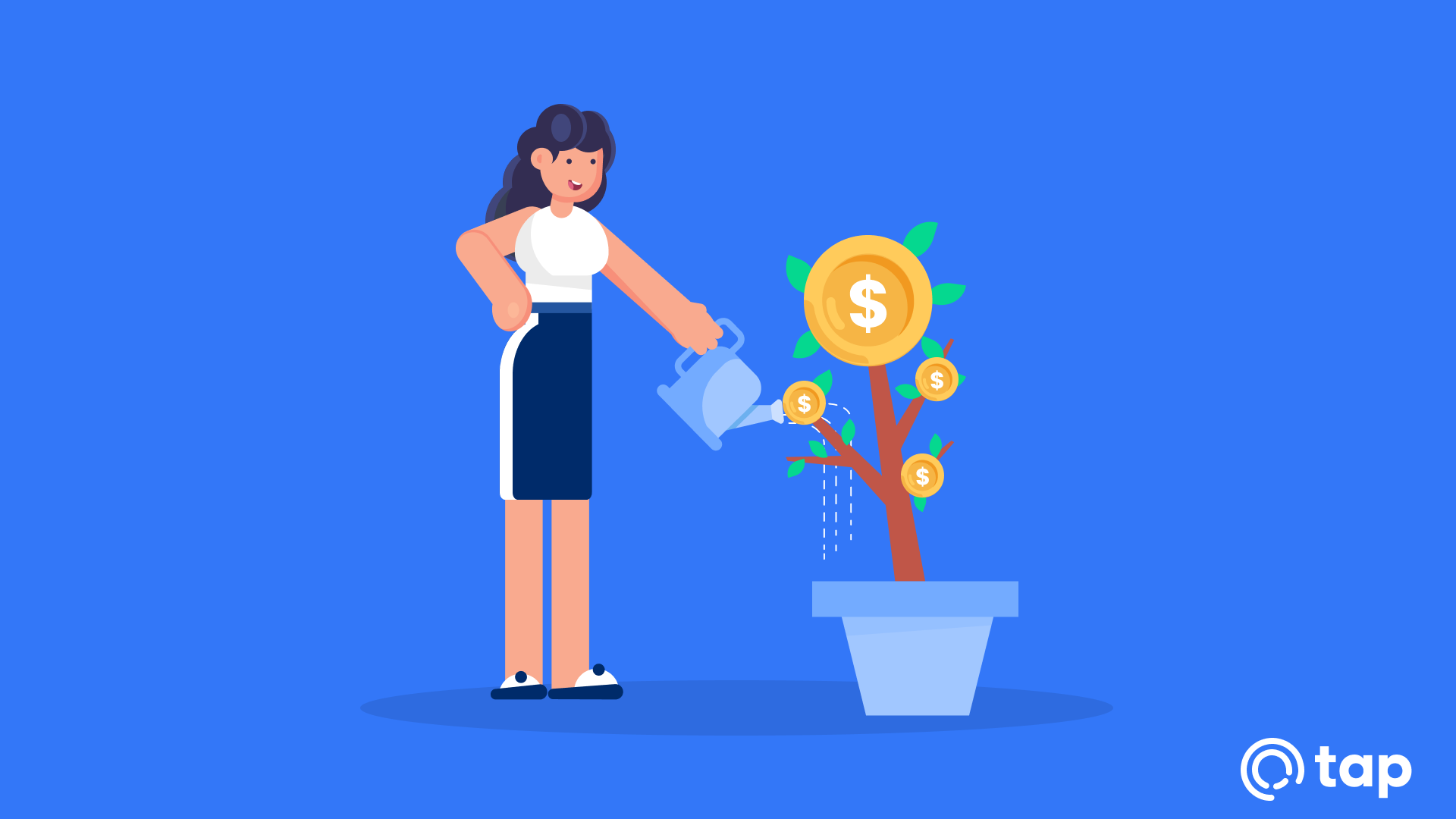# What Is A Return On Investment?

Investments are considered assets you buy in order to sell once the price of said asset has appreciated. So, what is a return on investment? Return on investment, or ROI, has come to be a universally accepted method to measure profitability.

ROI is calculated using a formula to work out your net return. You would take your latest asset value or price and subtract your initial investment value, this would be your net return. You would then divide your net return by the initial investment value, and multiply that total by 100.

An example would be an investment with a profit of \$100 and a cost of \$100 would have an ROI of x1, with a final investment value of \$200. With an initial investment of \$100 with a final investment value of \$300, you would have an ROI of x2, or 200%.

ROI is used to calculate how many times you have made your initial investment value back. Doubling your investment would give you an ROI of x1. In the cryptocurrency world for example, you will commonly hear cryptocurrency traders and investors talking about their ROI of “x10 or more”, it’s to refer to their return on investment.